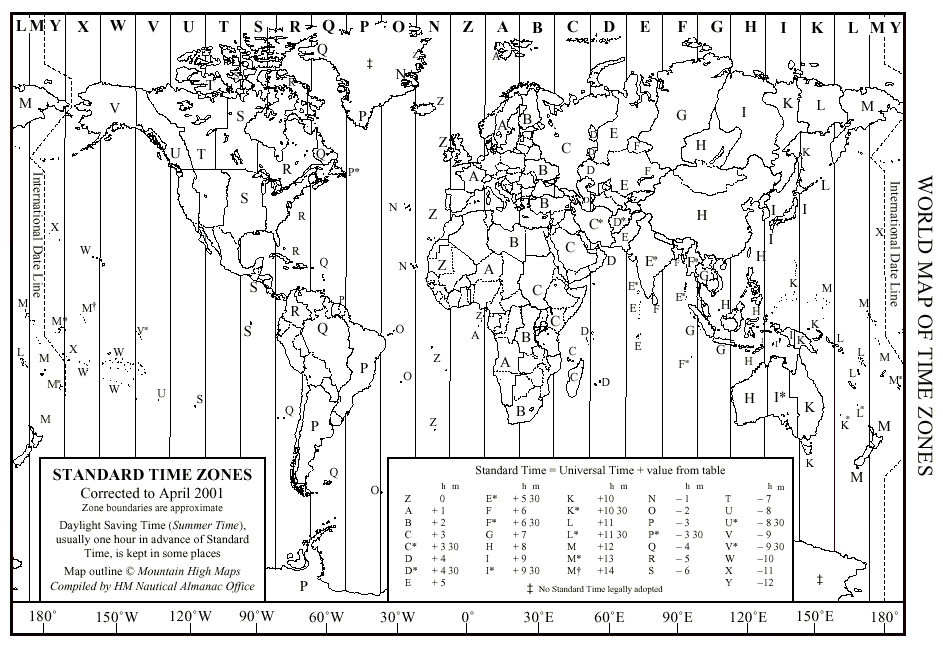The hour angle of the mean sun depends on the location of the observer and is different at different longitudes. It is therefore more convenient and less ambiguous to refer all times to a standard longitude, which was chosen by international agreement in 1884 to be the longitude of the old Royal Observatory at Greenwich. We can then define Greenwich mean time (GMT), or universal time (UT), in terms of the hour angle of the mean sun at Greenwich (GHAMS):

UT = GMT = GHAMS +/- 12h

the plus or minus sign being used if the GHAMS is less than or greater than 12h, respectively, in order to make the UT 0h at midnight. UT is related to the hour angle of the real Sun at Greenwich, GHAS, and the equation of time, E, by the relation:

UT = GHAS - E +/- 12h

For convenience, time within a particular country or geographical region is defined by time zones, 15° wide in longitude, within each of which the time is the same and approximates to the mean solar time at the centre of the zone. The standard time (ST) or zone time (ZT) is then defined by:

ST = ZT = UT + n

where n is given in hours and is a constant for a particular time zone, being negative for western longitudes and positive for eastern ones:

n = (longitude in degrees at centre of zone) / 15

In most cases n is taken to be an integer, so that different time zones are an exact number of hours apart. An exception is India, for example, where n=5.5. For geographical reasons, the time-zone borders do not always follow lines of longitude; for example, the International Date Line, which marks the centre of the zones +/-12, takes large excursions around groups of Pacific islands so that all islands in the group agree on the date. A world map of the time zones is shown in Figure 24.

 figure 24: World map of time zones.Before the advent of accurate clocks, the rotation of the Earth was the fundamental timekeeper for all purposes, both astronomical and civil. As soon as clocks became precise enough to detect fluctuations in the rotation rate of the Earth, the importance of astronomical observations for civil timekeeping began to diminish and nowadays intervals of time are defined by atomic clocks, the corresponding time scale being known as coordinated universal time (UTC). This drifts slowly with respect to UT, because of fluctuations in the Earth's rotation rate, and leap seconds are added from time to time to keep UTC in phase with UT (or else (civil) noon would eventually be at (astronomical) midnight). Thus we still rely on astronomical observations to keep the zero-point of our timescales correct.